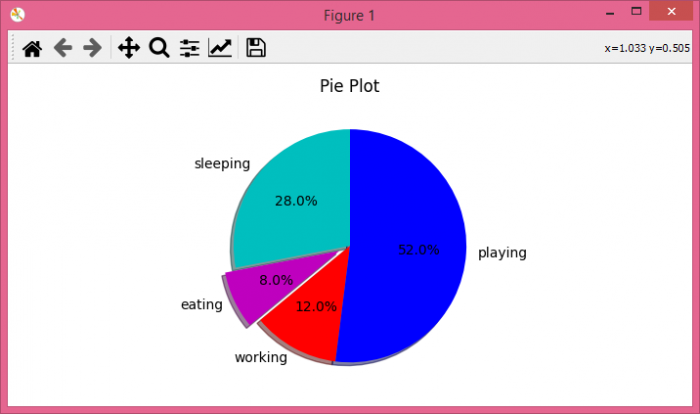# In Matplotlib, show the percentage or proportional data where each slice of pie represents a category

In this article, we can create a pie chart to show our daily activities, i.e., sleeping, eating, working, and playing. Using plt.pie() method, we can create a pie chart with the given different data sets for different activities.

## Steps

• Create a list of days, i.e., [1, 2, 3, 4, 5]. Similarly, make lists for sleeping, eating, playing, and working. There is an activities list that keeps “sleeping”, “eating”, “working” and “playing”.

• Make a list of colors.

• Use plt.pie() method to draw the pie chart, where slices, activities, colors as cols, etc. are passed.

• Set a title for the axes, i.e., “Pie Chart”.

• To show the figure use plt.show() method.

## Example

import matplotlib.pyplot as plt

days = [1, 2, 3, 4, 5]

sleeping = [7, 8, 6, 11, 7]
eating = [2, 3, 4, 3, 2]
working = [7, 8, 7, 2, 2]
playing = [8, 5, 7, 8, 13]
slices = [7, 2, 3, 13]
activities = ['sleeping', 'eating', 'working', 'playing']
cols = ['c', 'm', 'r', 'b']

plt.pie(slices,
labels=activities,
colors=cols,
startangle=90,
explode=(0, 0.1, 0, 0),
autopct='%1.1f%%')

plt.title('Pie Plot')
plt.show()

## Output

When we execute the code, it will produce the following output −